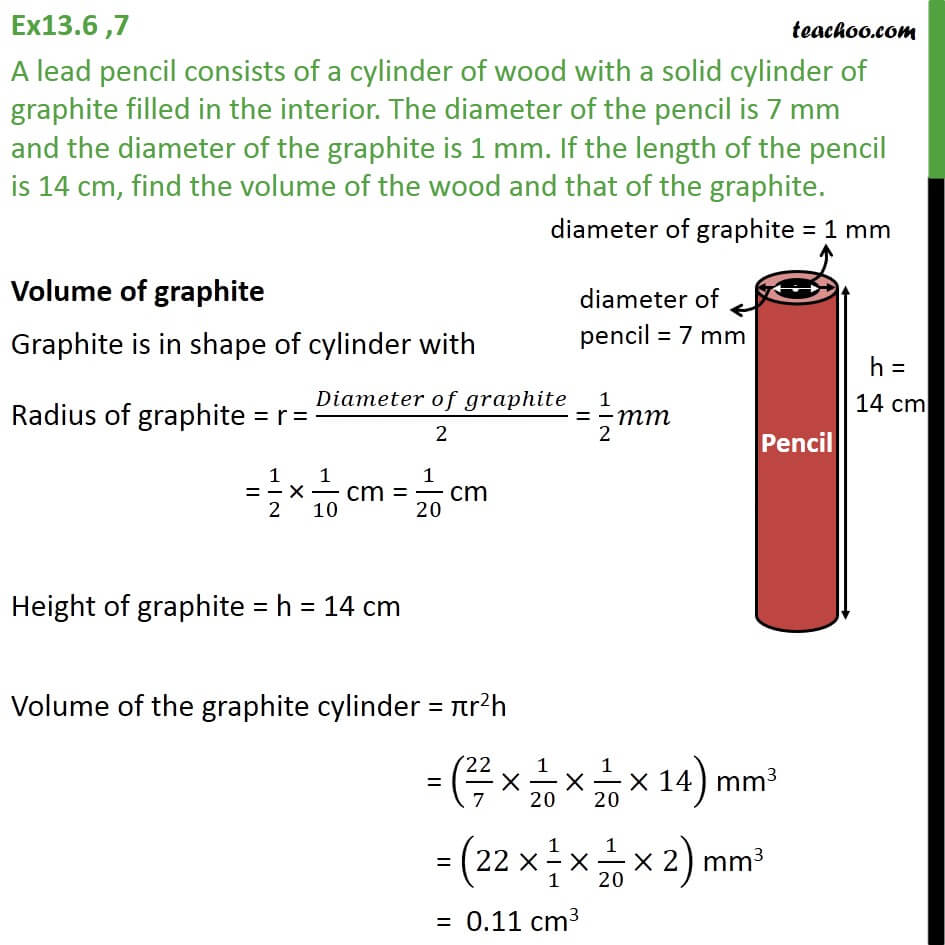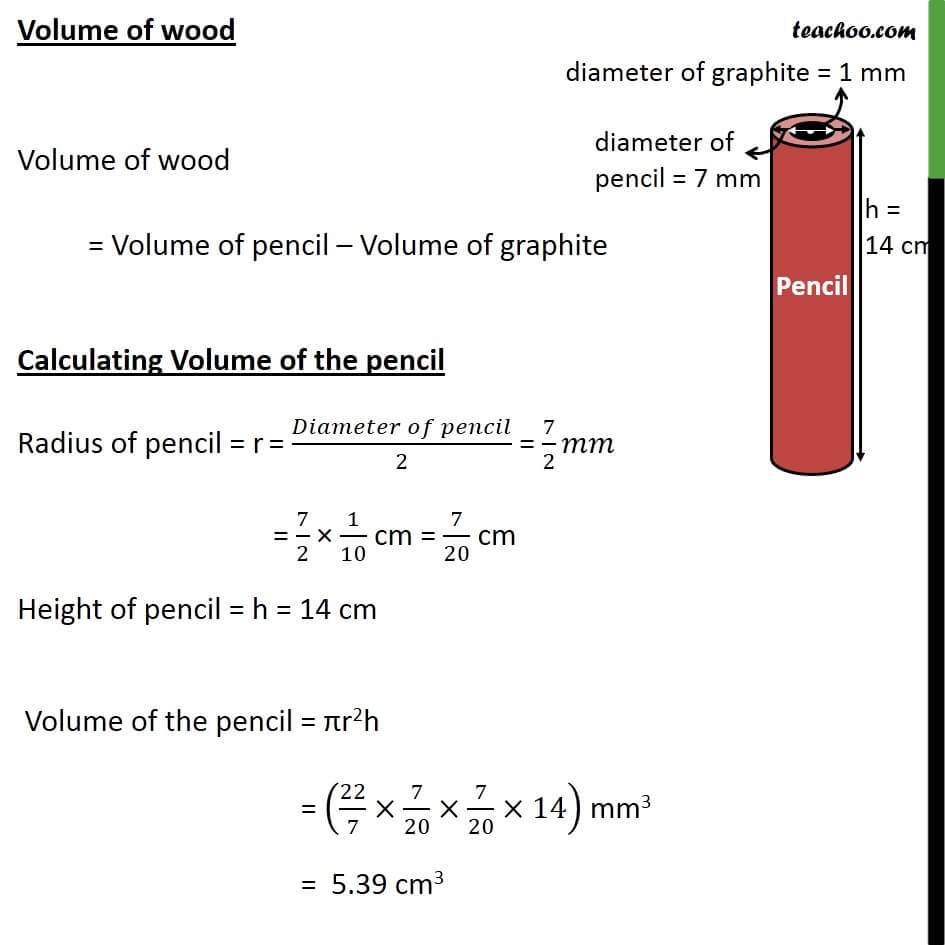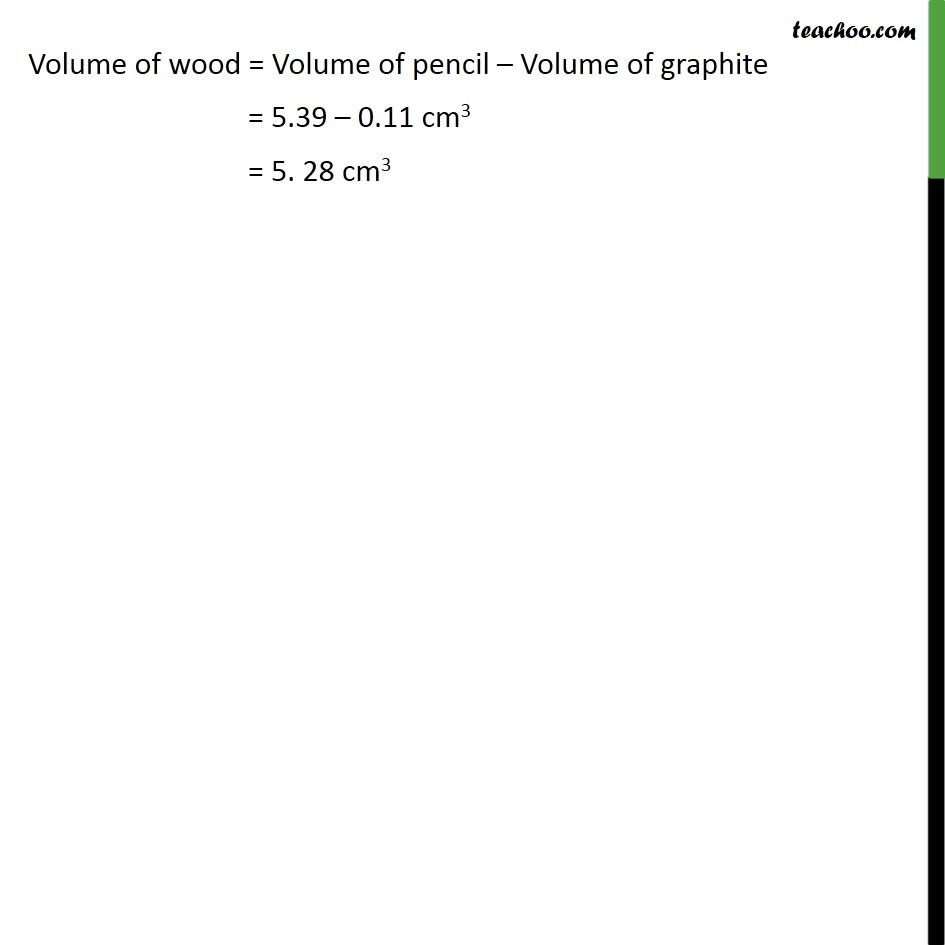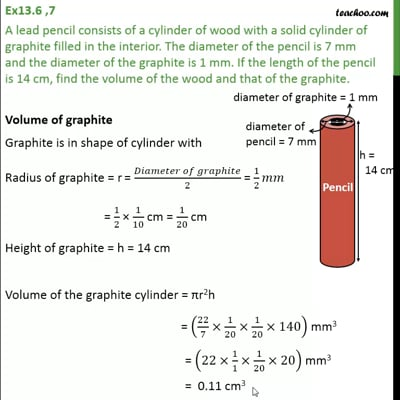Volume Of Cylinder

Chapter 13 Class 9 Surface Areas and Volumes
Concept wiseThis video is only available for Teachoo black users

Introducing your new favourite teacher - Teachoo Black, at only ₹83 per month

### Transcript

Ex13.6 ,7 A lead pencil consists of a cylinder of wood with a solid cylinder of graphite filled in the interior. The diameter of the pencil is 7 mm and the diameter of the graphite is 1 mm. If the length of the pencil is 14 cm, find the volume of the wood and that of the graphite. Volume of graphite Graphite is in shape of cylinder with Radius of graphite = r = (𝐷𝑖𝑎𝑚𝑒𝑡𝑒𝑟 𝑜𝑓 𝑔𝑟𝑎𝑝ℎ𝑖𝑡𝑒)/2 = 1/2 𝑚𝑚 = 1/2 × 1/10 cm = 1/20 cm Height of graphite = h = 14 cm Volume of the graphite cylinder = πr2h = (22/7×1/20×1/20×140) mm3 = (22×1/1×1/20×20) mm3 = 0.11 cm3 Volume of wood Volume of wood = Volume of pencil – Volume of graphite Calculating Volume of the pencil Radius of pencil = r = (𝐷𝑖𝑎𝑚𝑒𝑡𝑒𝑟 𝑜𝑓 𝑝𝑒𝑛𝑐𝑖𝑙)/2 = 7/2 𝑚𝑚 = 7/2 × 1/10 cm = 7/20 cm Height of pencil = h = 14 cm Volume of the pencil = πr2h = (22/7×7/20×7/20×140) mm3 = 5.39 cm3 Volume of wood = Volume of pencil – Volume of graphite = 5.39 – 0.11 cm3 = 5. 28 cm3